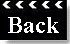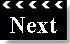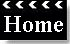SNOWFLAKE PATHWAYS

A HISTORY IN PICTURES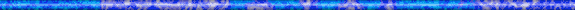Snow Pix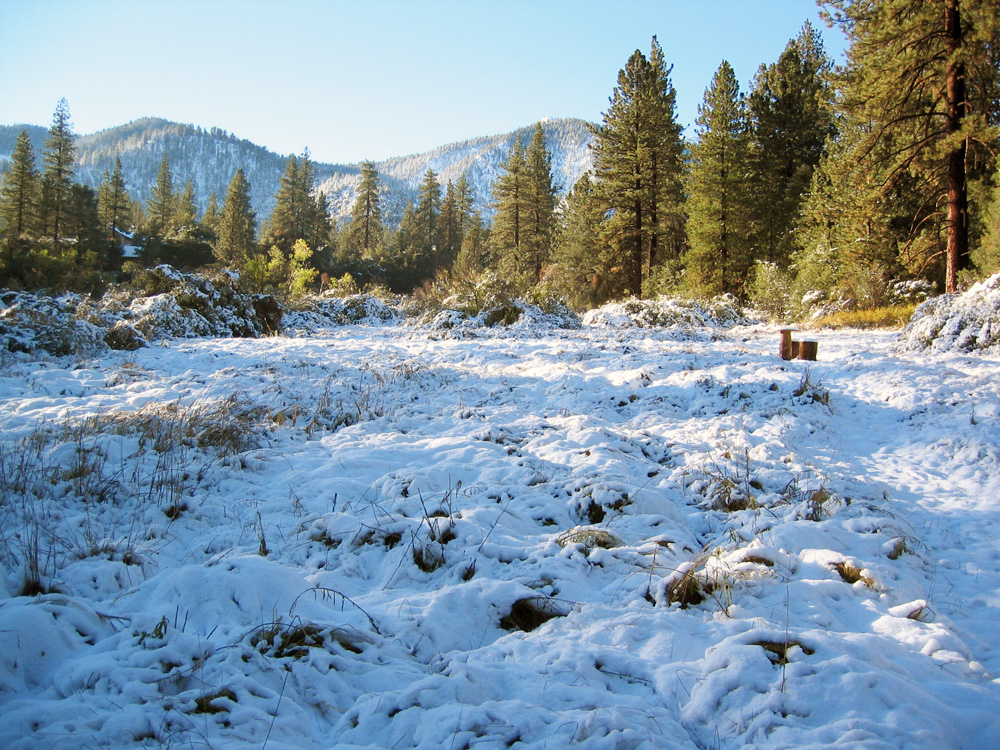(1) ^ ^ ^ ^ The wetlands meadow (October 27, 2004).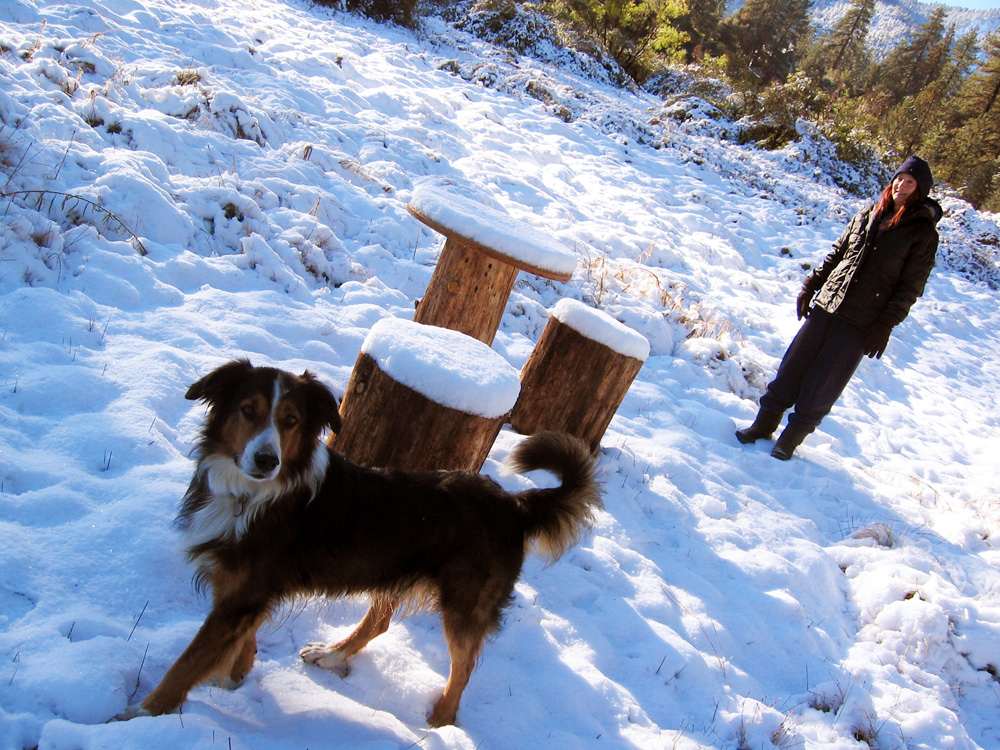(2) ^ ^ ^ ^ The Sunset Table in the wetlands meadow (October 27, 2004).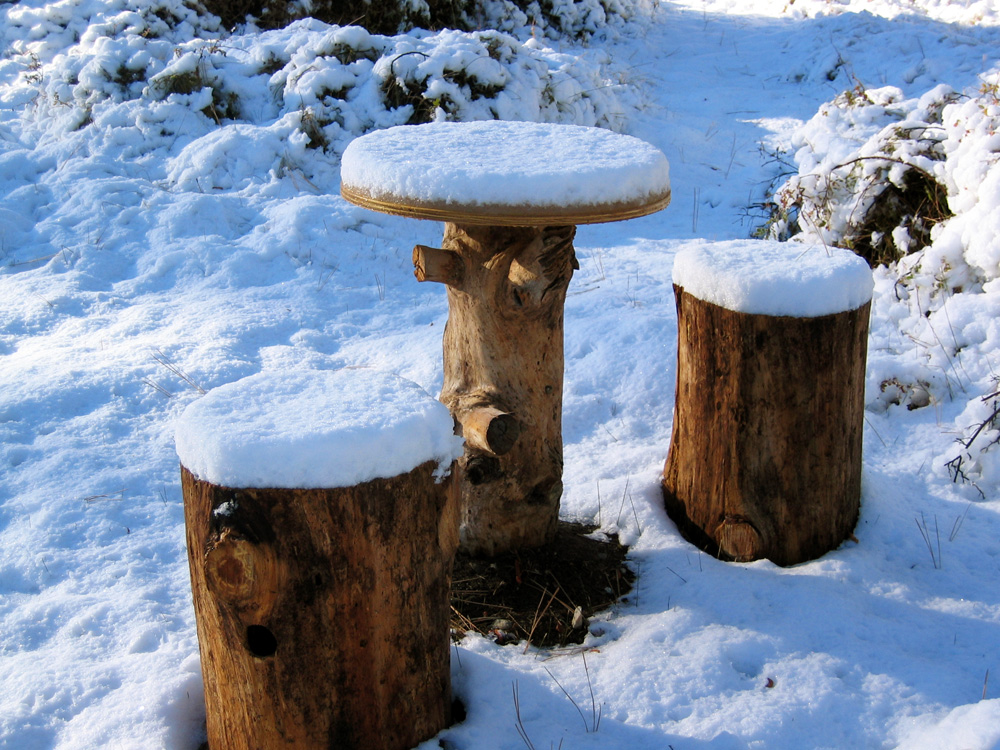(3) ^ ^ ^ ^ The Wild Roses Table (October 27, 2004).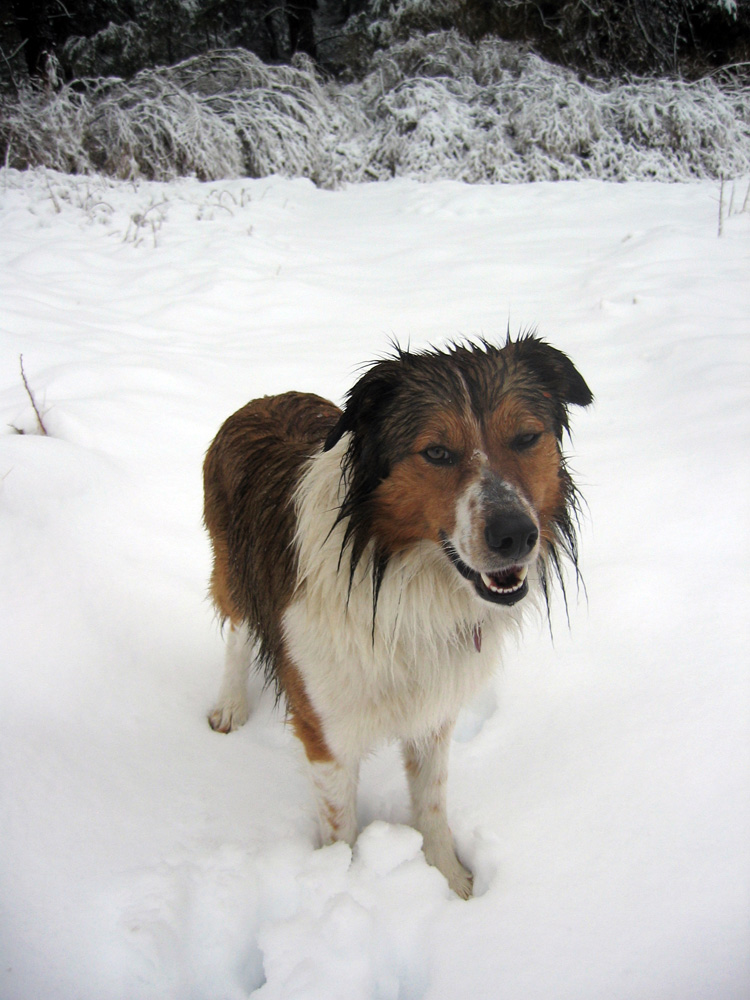(4) ^ ^ ^ ^ Max loves to play in the snow (December 28, 2004).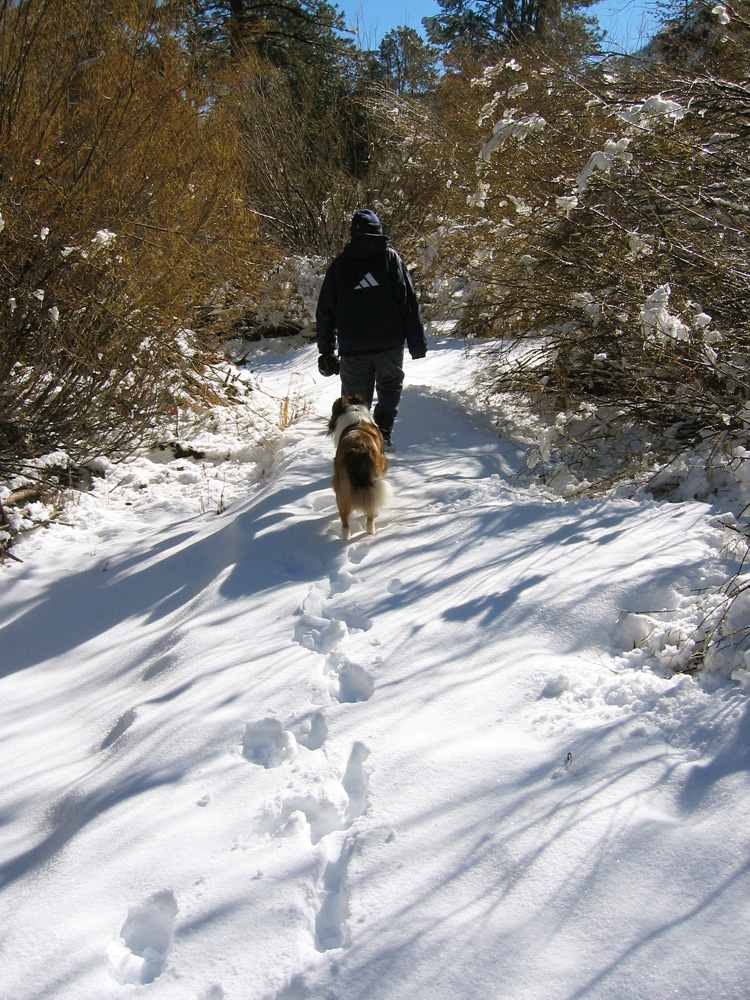(5) ^ ^ ^ ^ Wetlands Pathway heading east (January 30, 2005).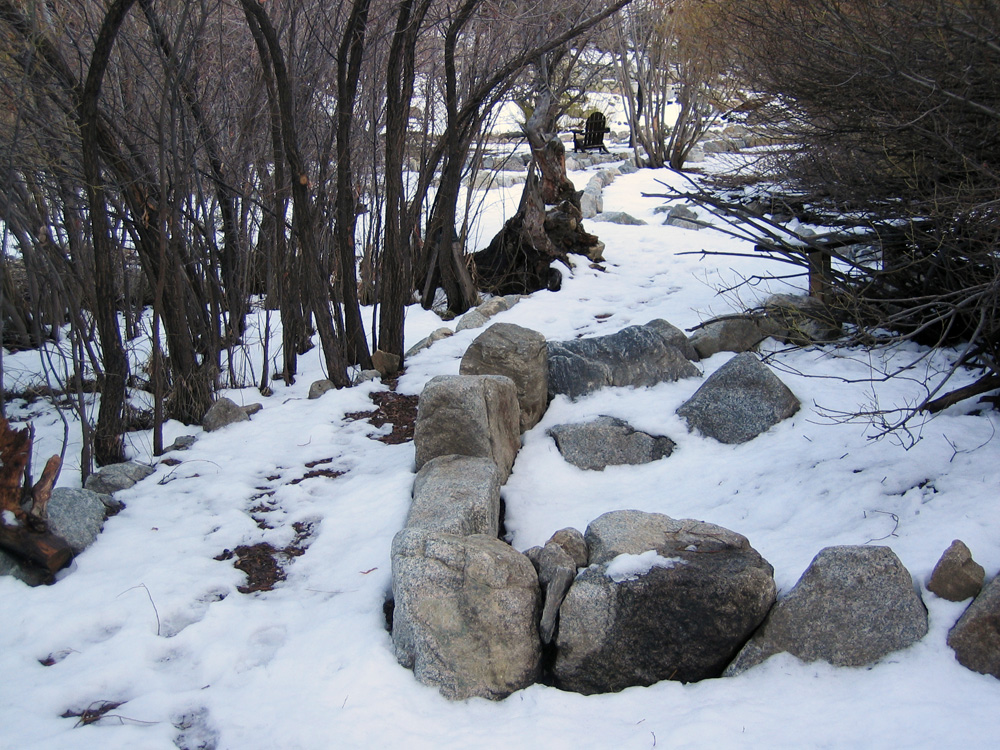(6) ^ ^ ^ ^ Wetlands Pathway looking east (February 1, 2005).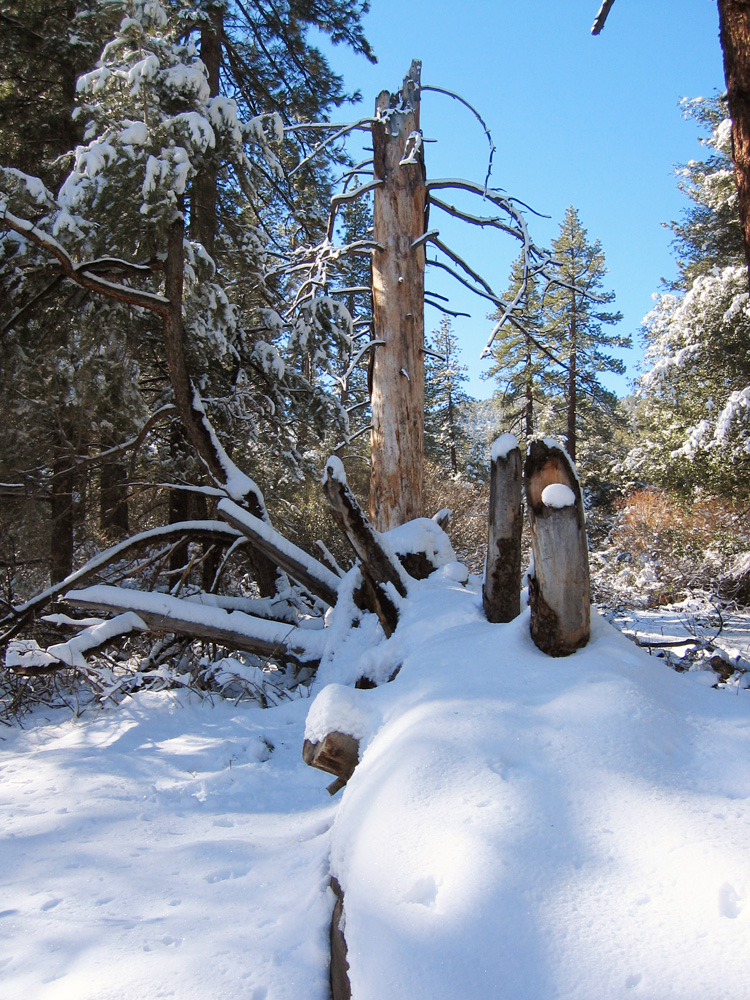(7) ^ ^ ^ ^ Snow covered log from an old fallen tree (January 30, 2005).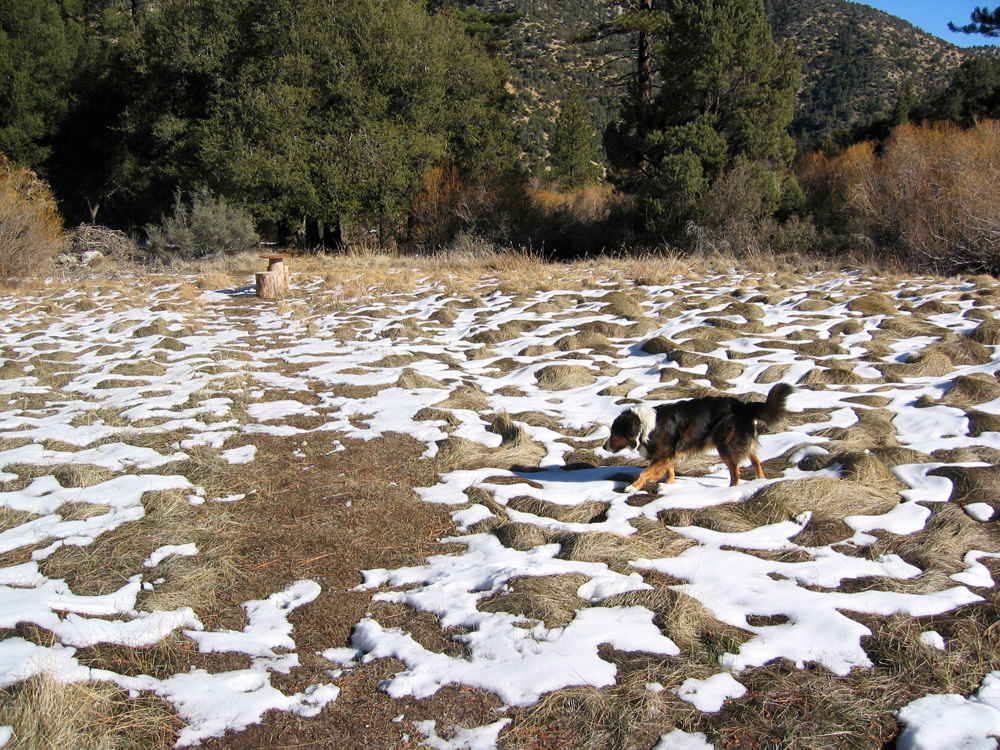(8) ^ ^ ^ ^ The wetlands meadow (February 2, 2005).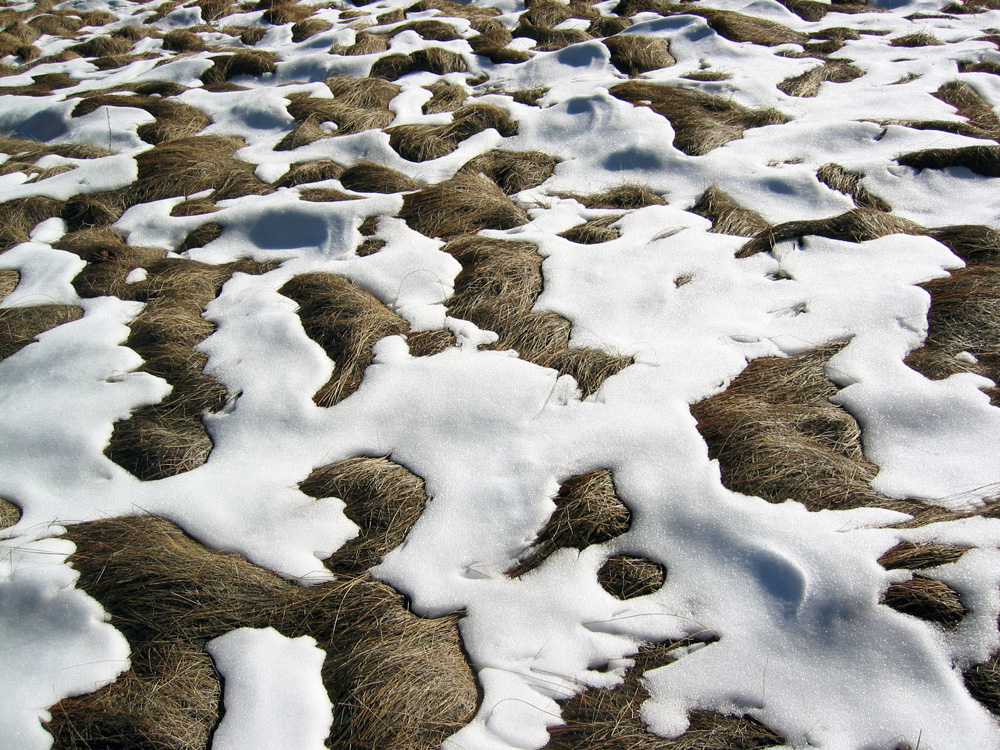(9) ^ ^ ^ ^ The wetlands meadow with melting snow (February 2, 2005).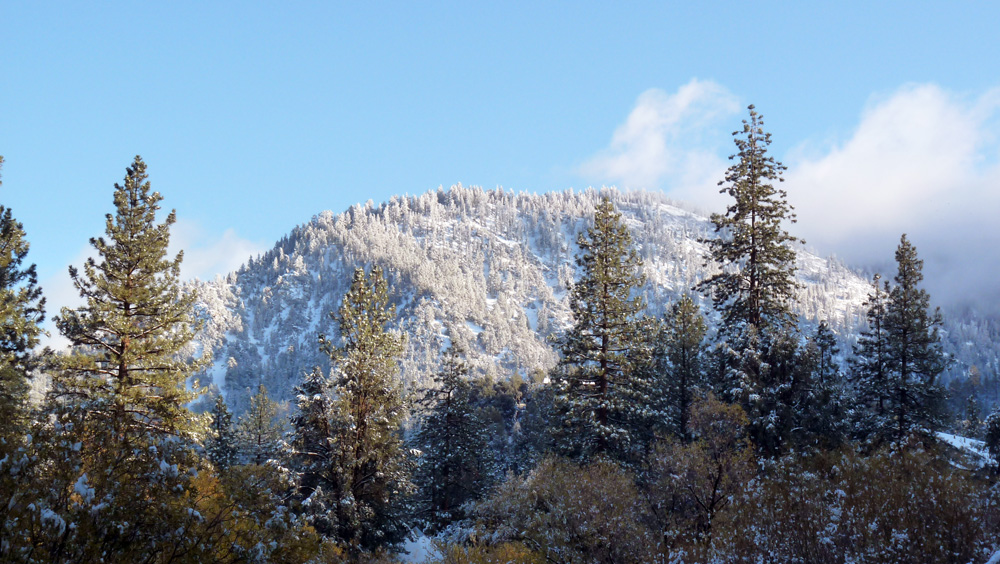(10) ^ ^ ^ ^ The slopes of Mount Pinos (the actual summit can not be seen from this vantage point).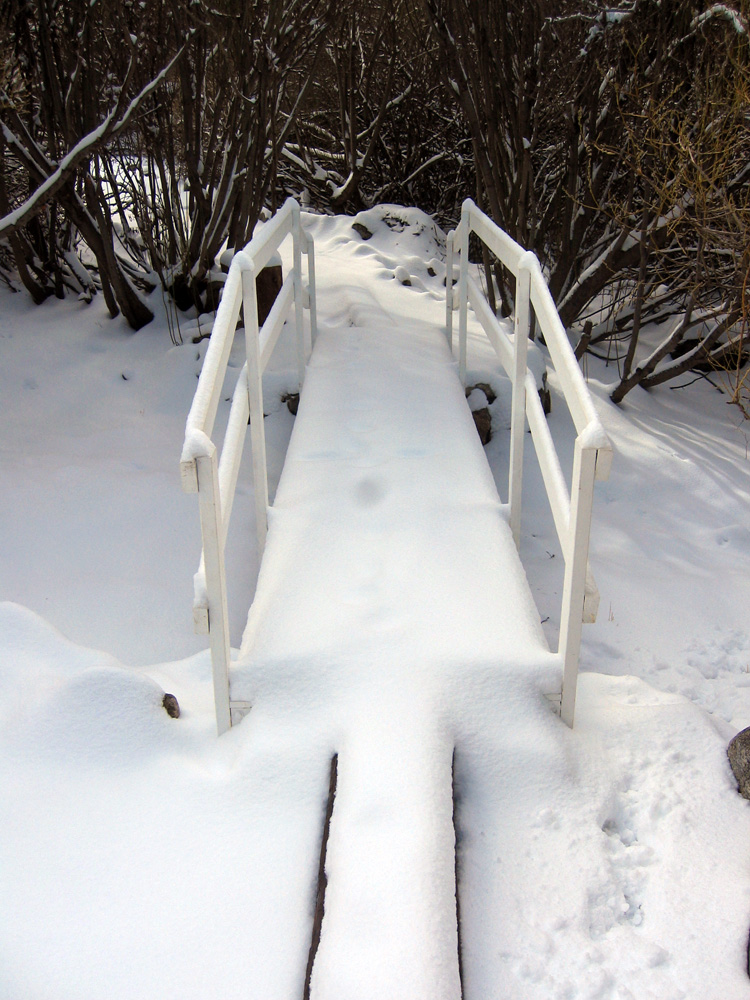(11) ^ ^ ^ ^ Wedding Bridge (March 10, 2006).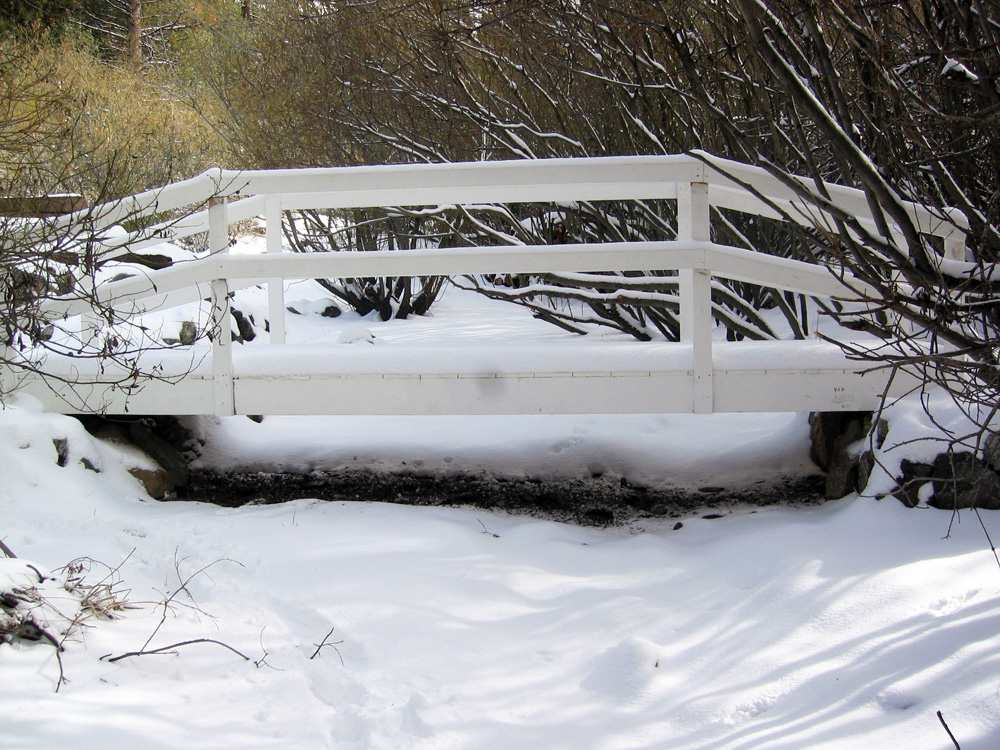(12) ^ ^ ^ ^ Wedding Bridge (March 10, 2006).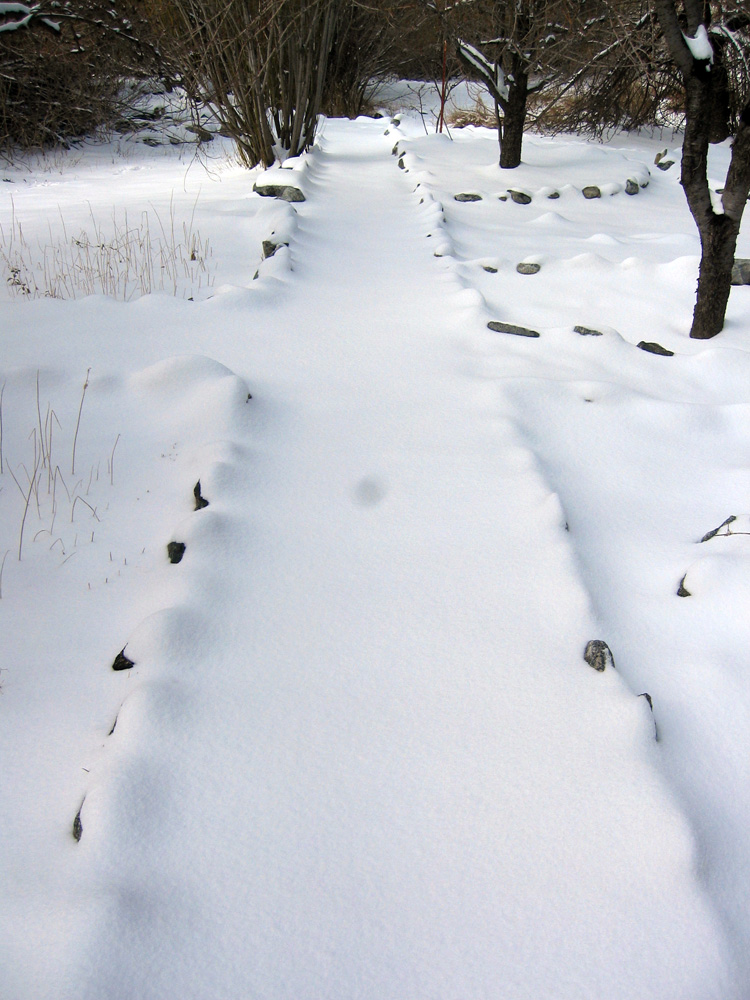(13) ^ ^ ^ ^ The start of the Wetlands Pathway heading west (March 10, 2006).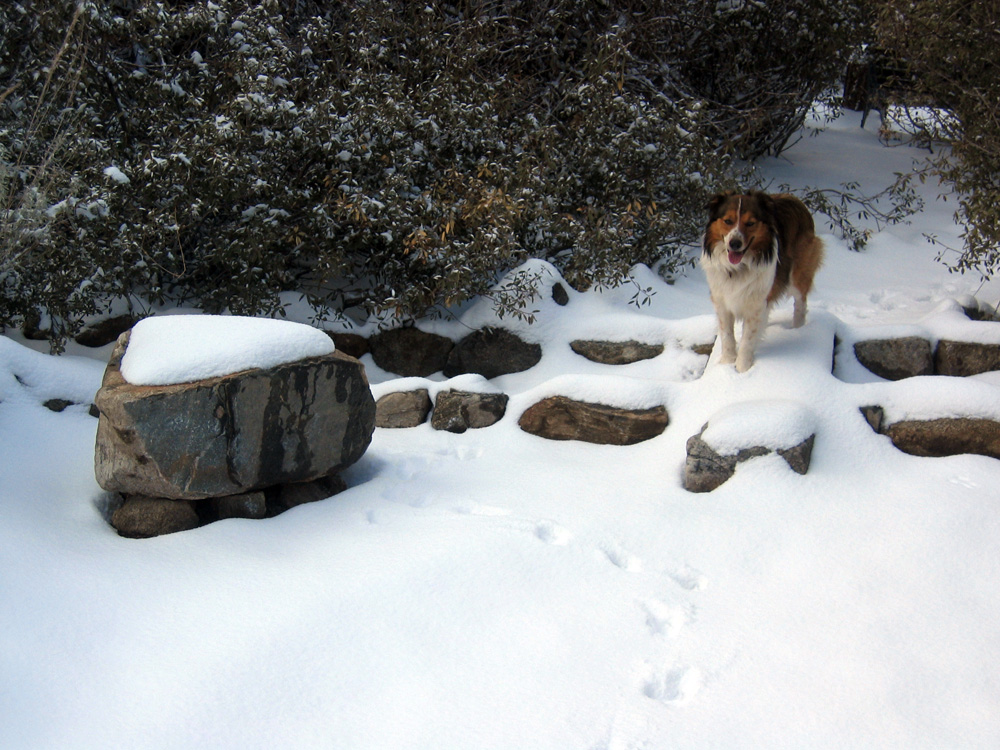(14) ^ ^ ^ ^ Zen Garden (March 10, 2006).(15) ^ ^ ^ ^ Zen Garden foot bridge (January 19,2006).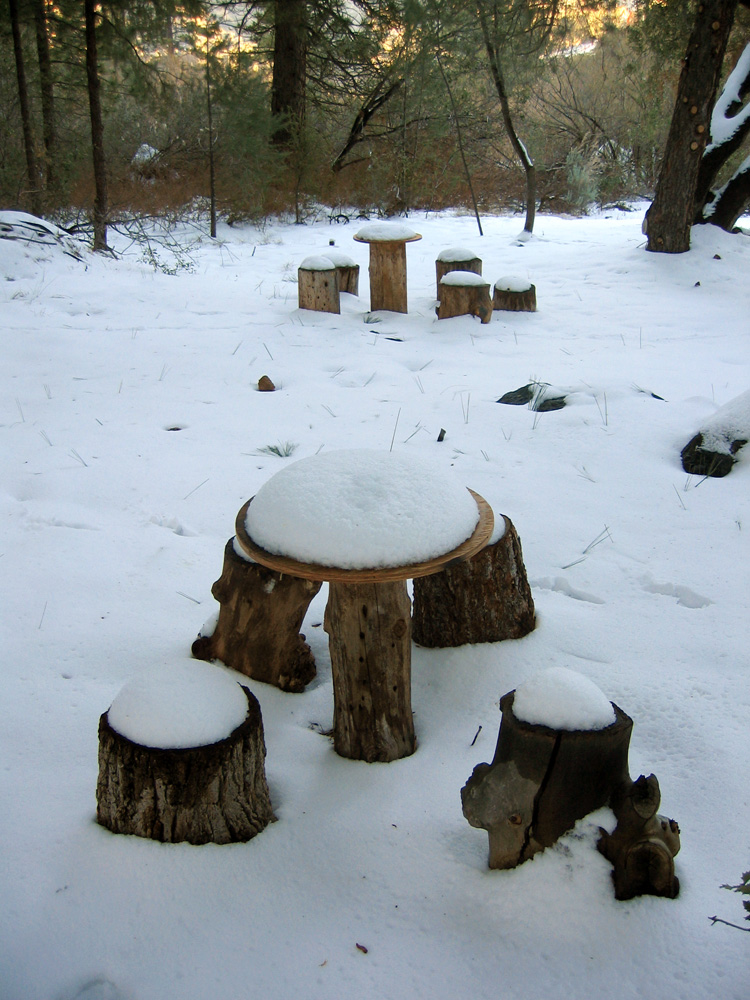(16) ^ ^ ^ ^ Enchanted Forest (March 13, 2006). The table in the foreground has since been relocated.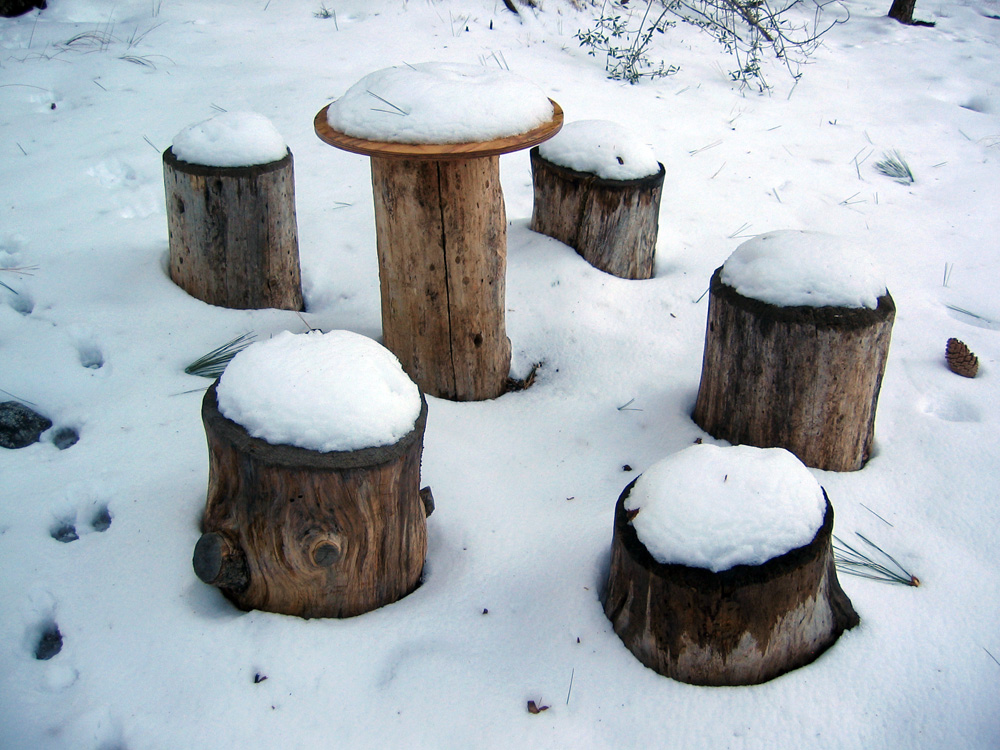(17) ^ ^ ^ ^ Enchanted Forest (March 13, 2006).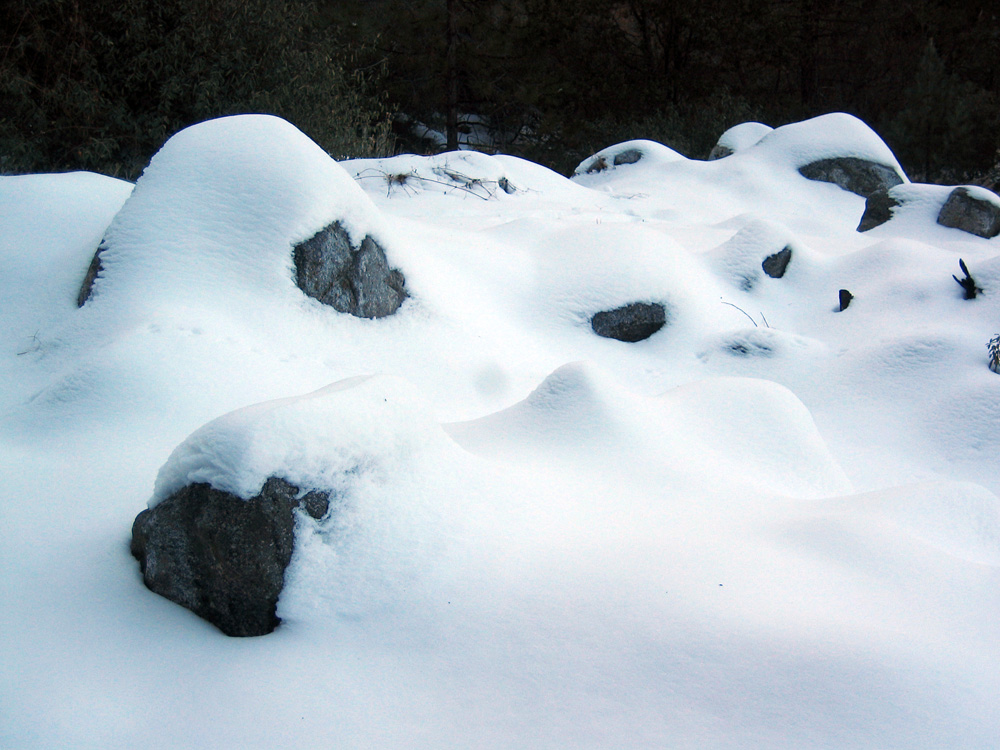(18) ^ ^ ^ ^ Snow mounds (March 13, 2006).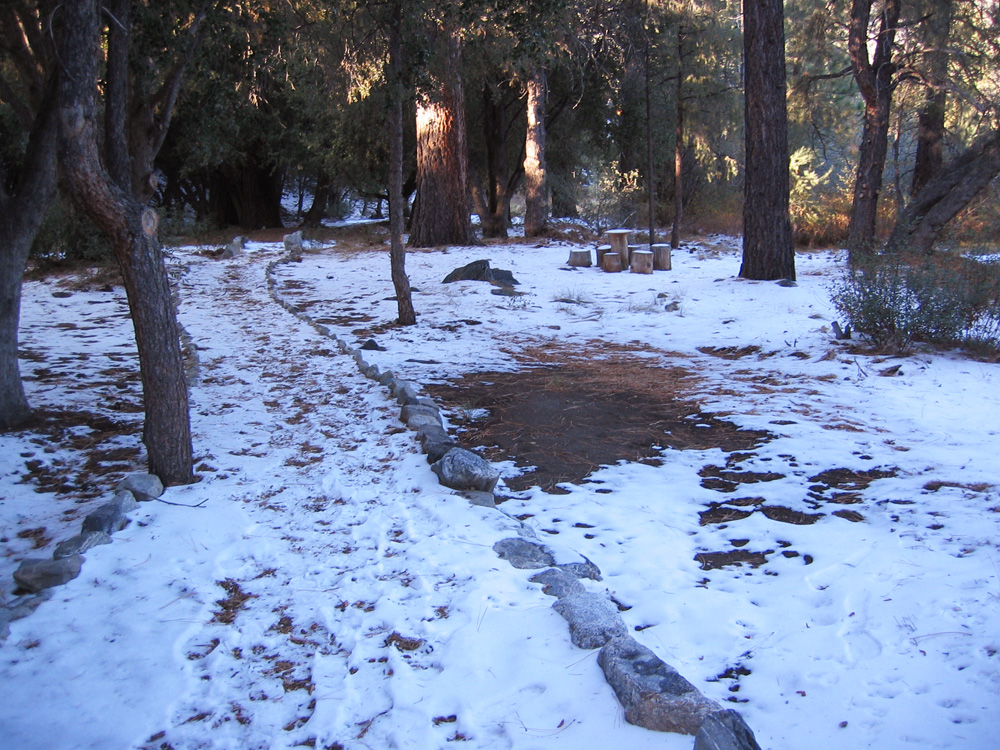(19) ^ ^ ^ ^ Enchanted Forest (December 13, 2007).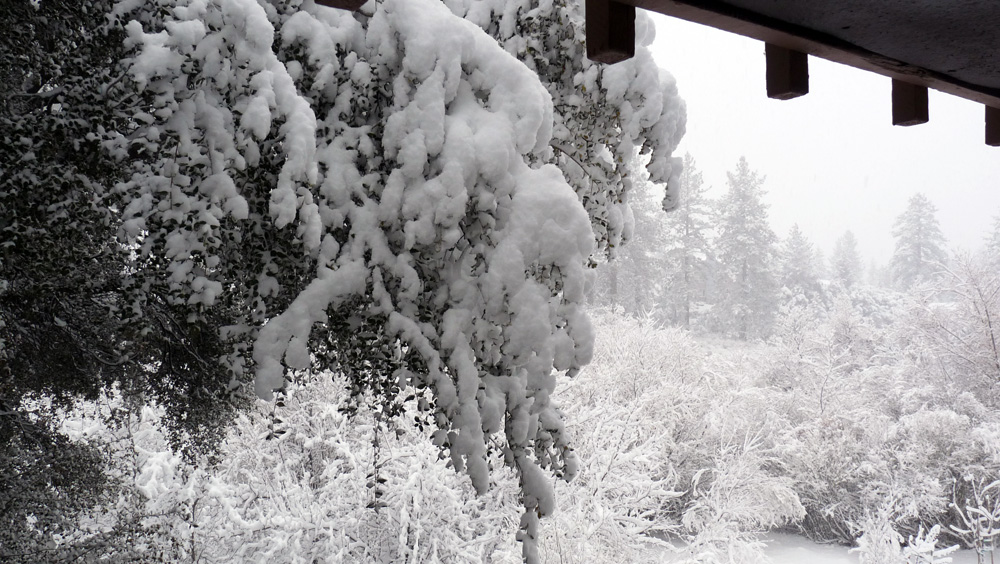(20) ^ ^ ^ ^ The green belt looking west (December 15, 2008).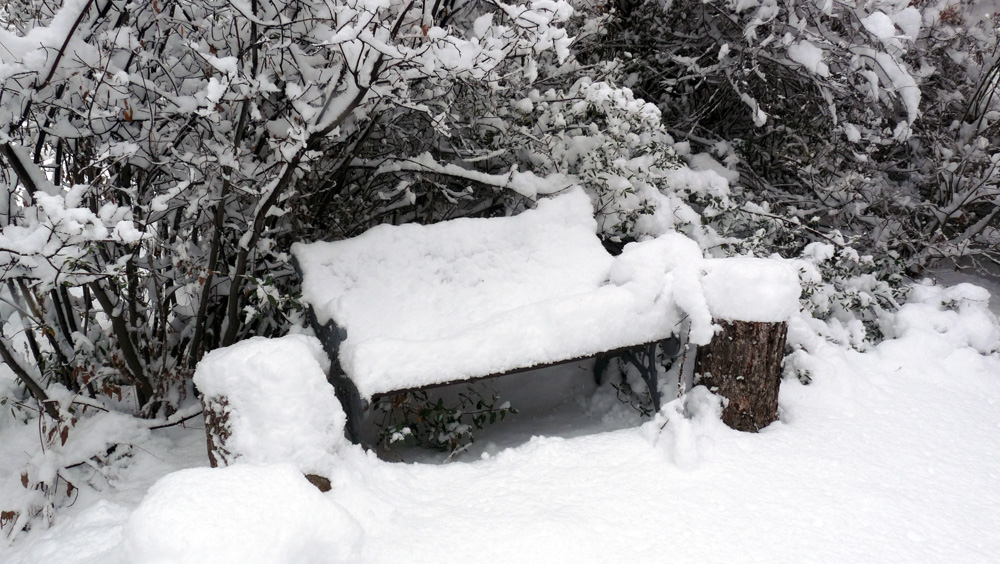(21) ^ ^ ^ ^ Bench (December 15, 2008).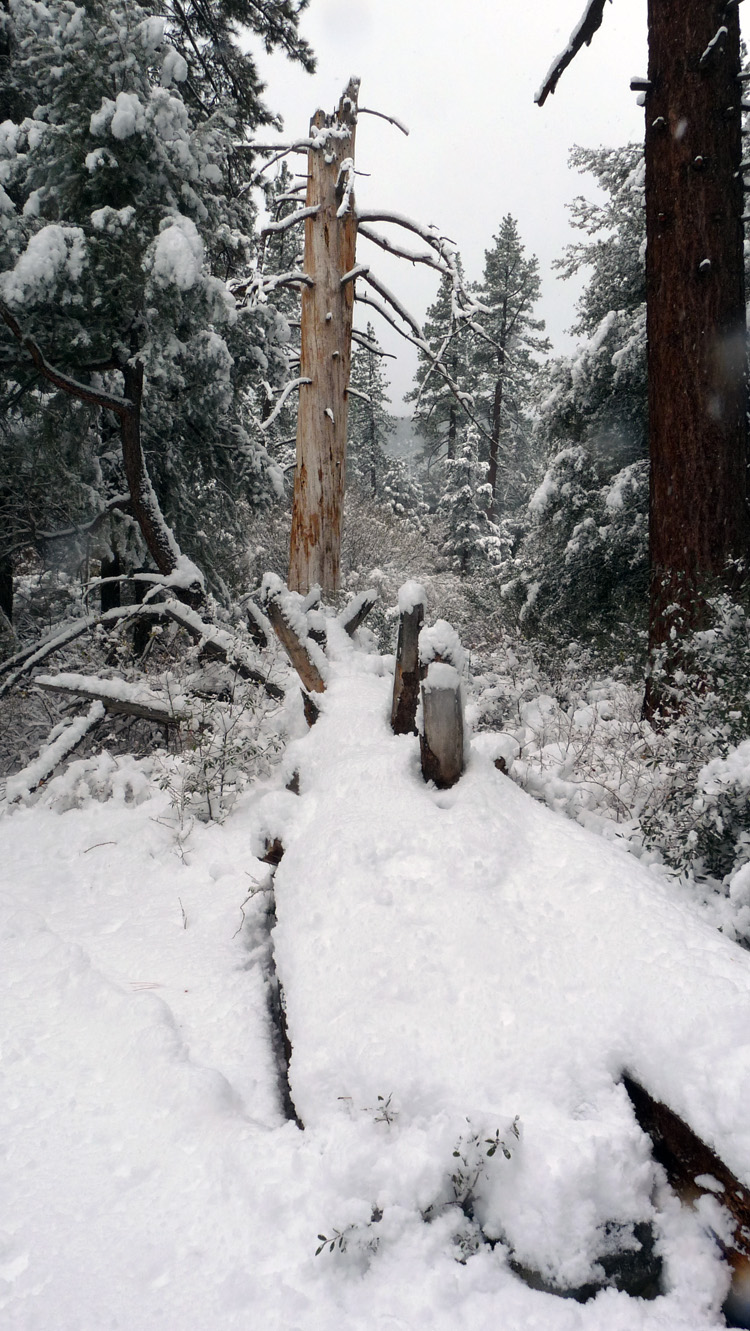(22) ^ ^ ^ ^ Snow covered log from a fallen tree (December 15, 2008).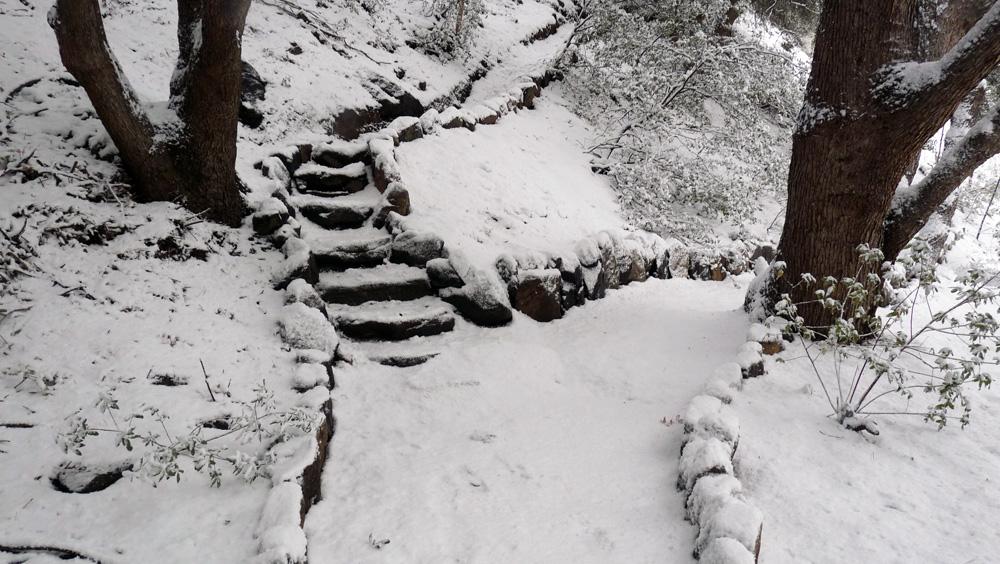(23) ^ ^ ^ ^ Flight of stone steps leading up to Freeman Drive (December 15, 2008).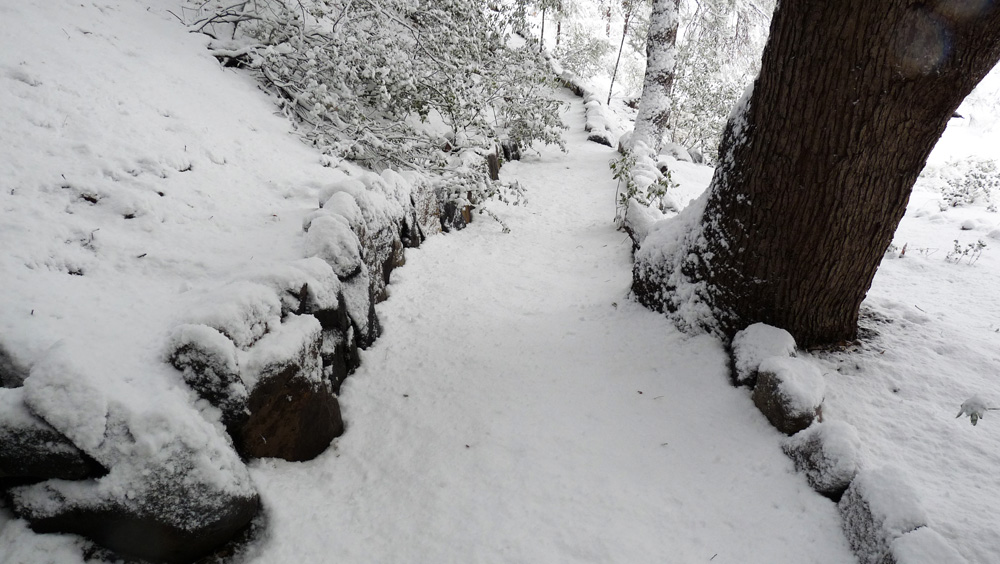(24) ^ ^ ^ ^ Alternative pathway going up to Freeman Drive (December 15, 2008).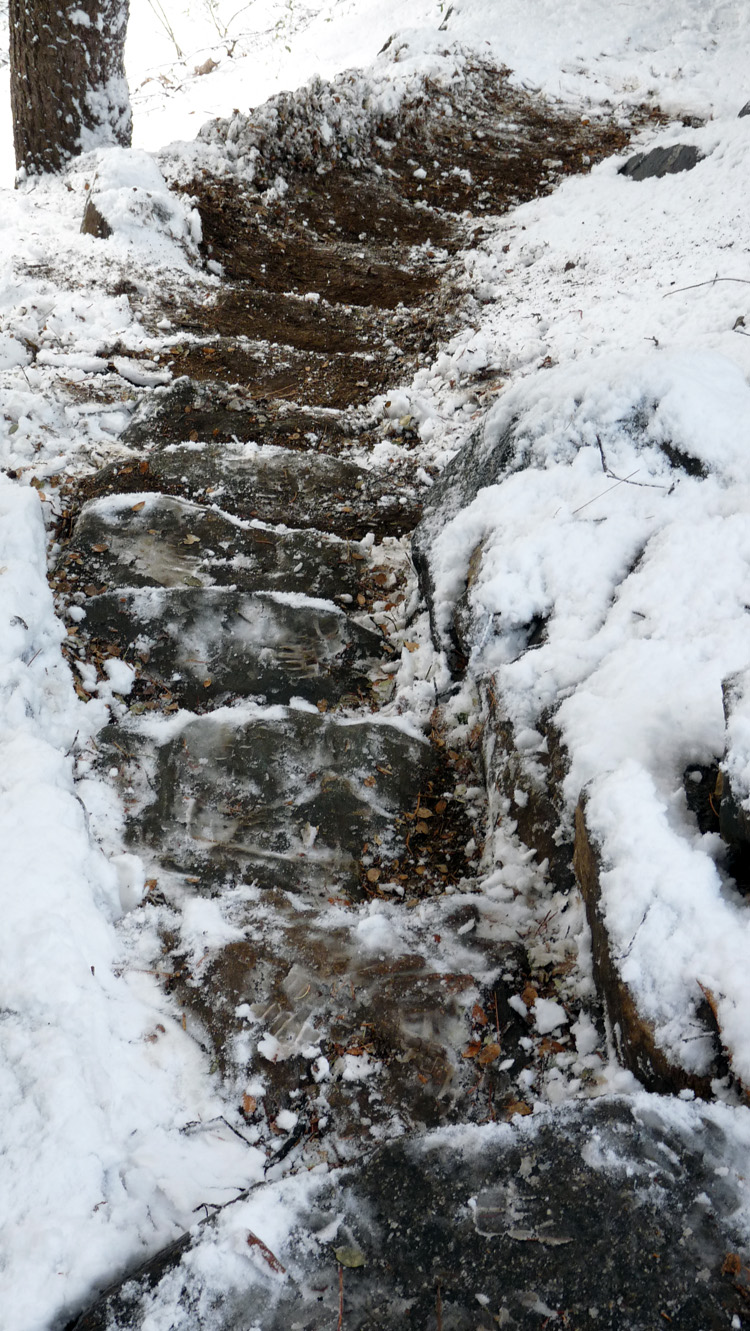(25) ^ ^ ^ ^ Stone steps near Freeman Drive cleared of snow (December 15, 2008).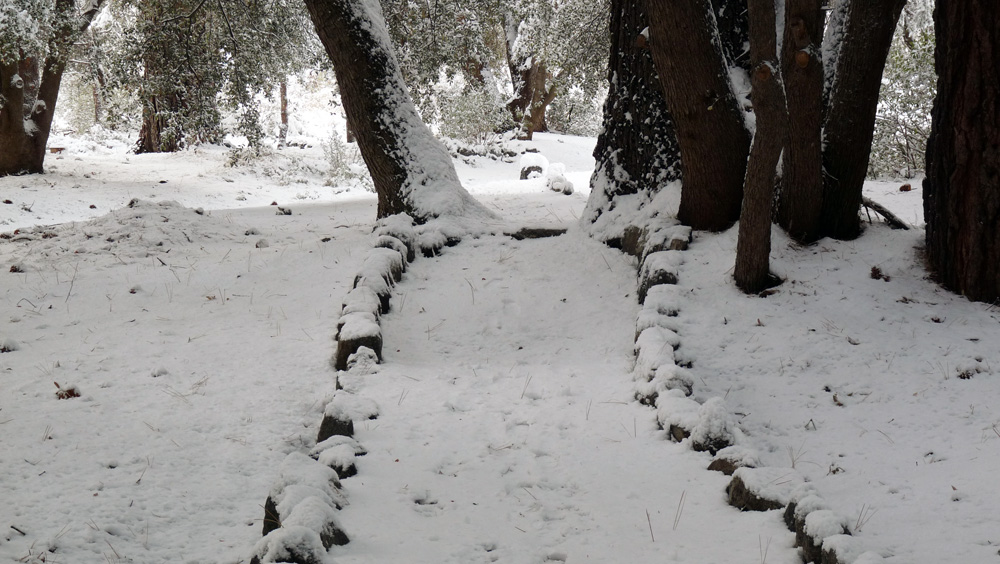(26) ^ ^ ^ ^ Approaching the Enchanted Forest (December 15, 2008).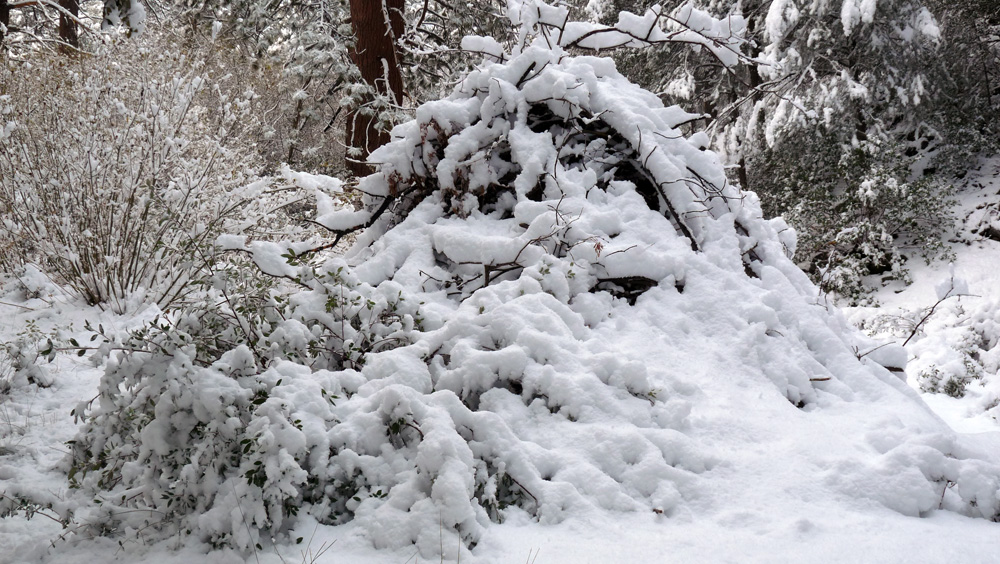(27) ^ ^ ^ ^ Pile from clearing work (December 15, 2008).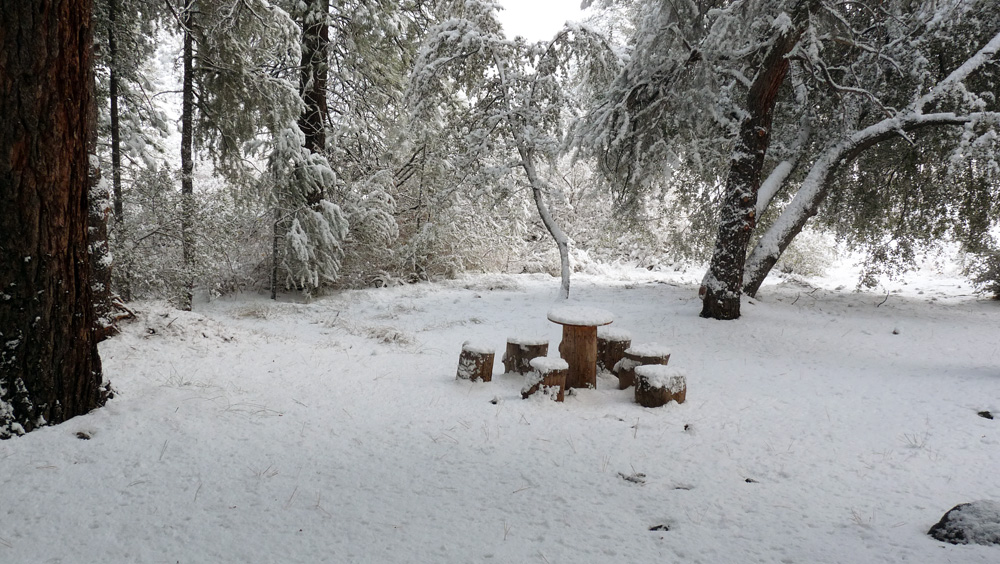(28) ^ ^ ^ ^ Enchanted Forest (December 15, 2008).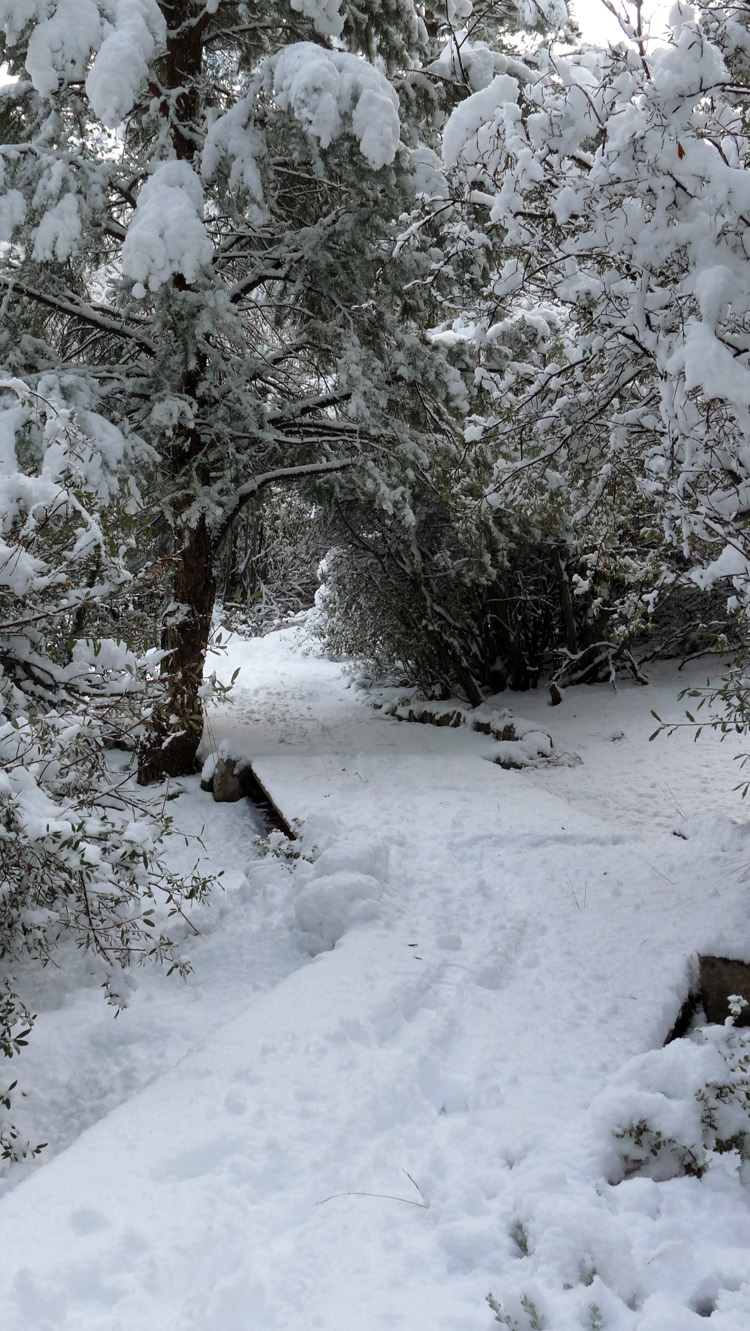(29) ^ ^ ^ ^ Snow covered foot bridges (December 15, 2008).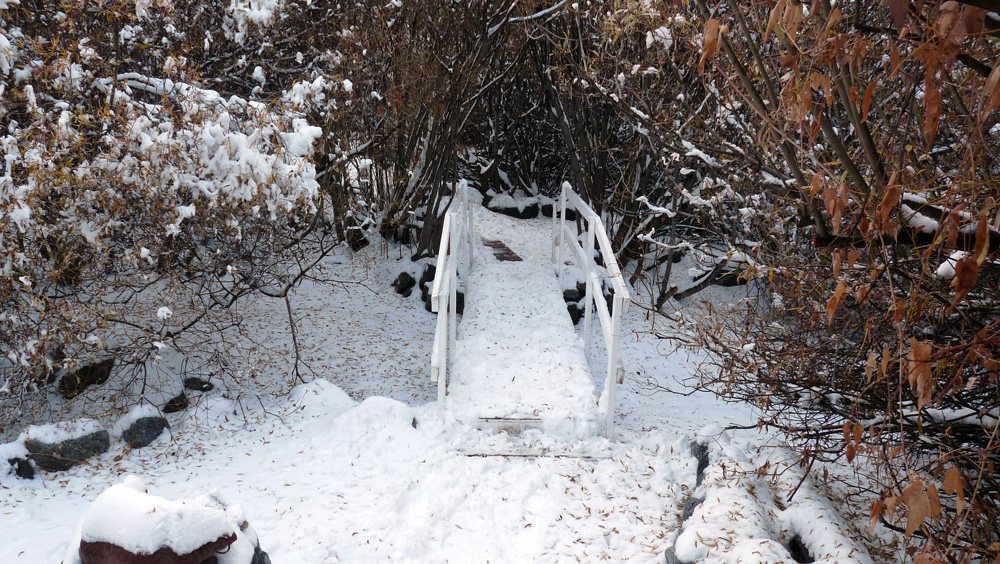(30) ^ ^ ^ ^ Wedding Bridge (November 28, 2009).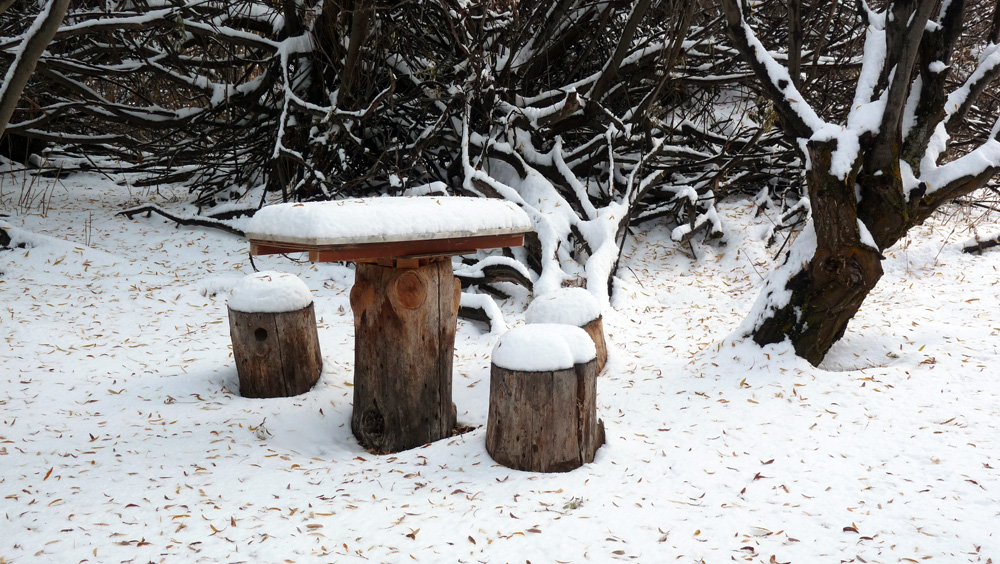(31) ^ ^ ^ ^ Marble Grove Table (November 28, 2009).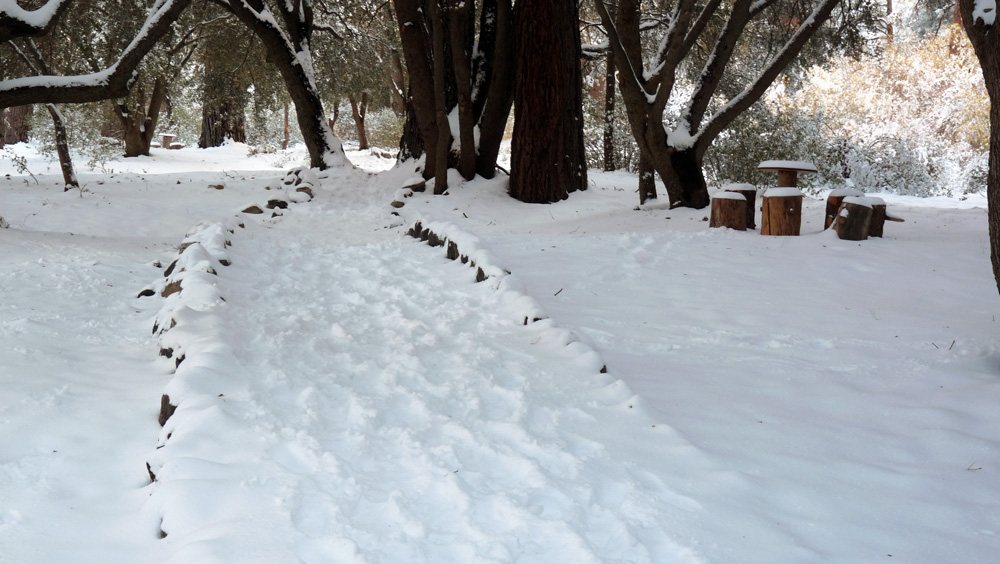(32) ^ ^ ^ ^ Upper Oak Grove Table (November 28, 2009).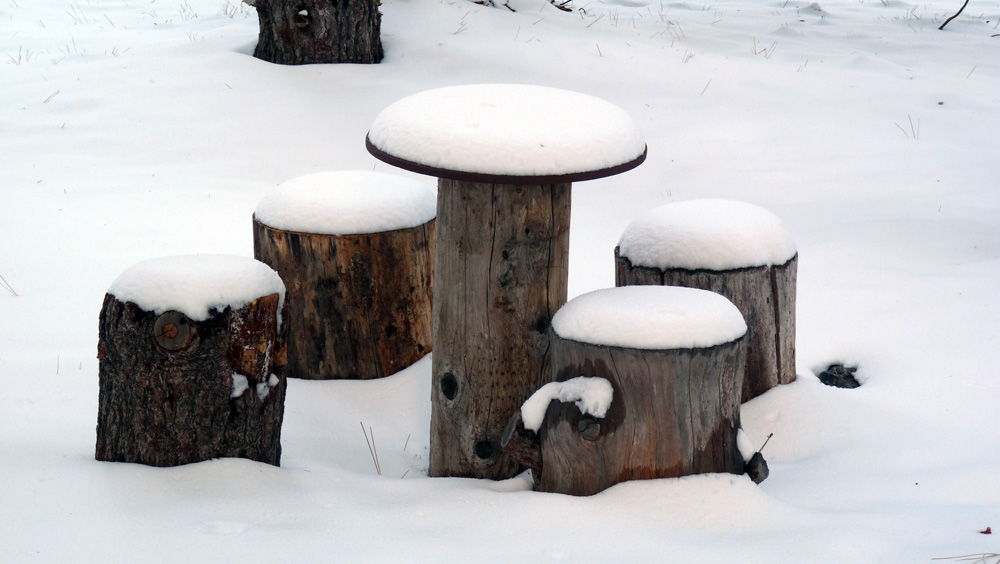(33) ^ ^ ^ ^ Enchanted Forest (November 28, 2009).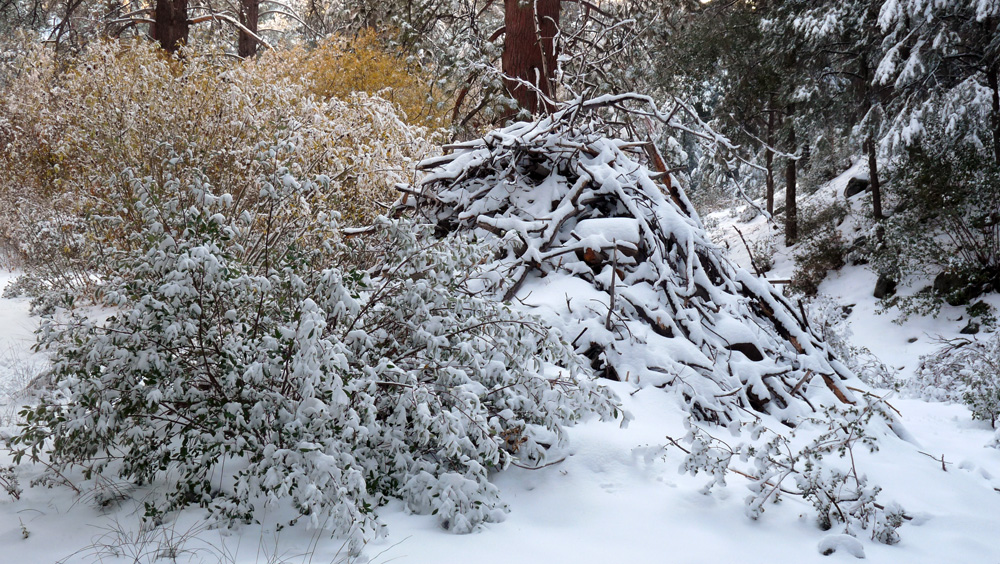(34) ^ ^ ^ ^ Pile from clearing work (November 28, 2009).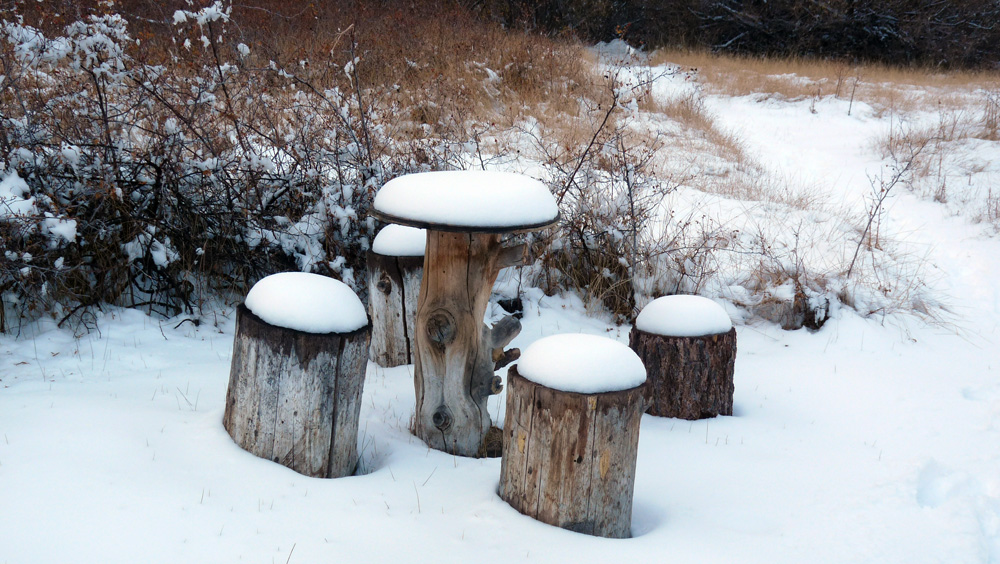(35) ^ ^ ^ ^ Wild Roses Table (November 28, 2009).